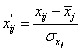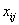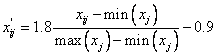Home News About Me Ph. D. Thesis Abstract Table of Contents 1. Introduction 2. Theory  Fundamentals of the Multivariate Data Analysis 2.1. Overview of the Multivariate Quantitative Data Analysis 2.2. Experimental Design 2.3. Data Preprocessing 2.4. Data Splitting and Validation 2.5. Calibration of Linear Relationships 2.6. Calibration of Nonlinear Relationships 2.7. Neural Networks  Universal Calibration Tools 2.8. Too Much Information Deteriorates Calibration 2.9. Measures of Error and Validation 3. Theory  Quantification of the Refrigerants R22 and R134a: Part I 4. Experiments, Setups and Data Sets 5. Results  Kinetic Measurements 6. Results  Multivariate Calibrations 7. Results  Genetic Algorithm Framework 8. Results  Growing Neural Network Framework 9. Results  All Data Sets 10. Results  Various Aspects of the Frameworks and Measurements 11. Summary and Outlook 12. References 13. Acknowledgements Publications Research Tutorials Downloads and Links Contact Search Site Map Print this Page## 2.3.   Data Preprocessing

Data preprocessing can be used for systematically modifying the raw signals of the device with the hope that the altered signals provide more useful input to the calibration method. Unfortunately, no general guidelines exist to determine the appropriate data preprocessing technique and thus the different preprocessing techniques are controversially discussed in literature ,.

In this work, the input variables are preprocessed by autoscaling according to:(2)

Withas response of the ith sample at the jth variable,as the mean of the jth variable andas the standard deviation of the jth variable. Autoscaling involves a mean-centering of the data and a division by the standard deviation of all responses of a particular input variable resulting in a mean of zero and a unit standard deviation of each variable. For some calibration methods autoscaling can improve the calibration as autoscaling allows all variables to influence equally the calibration especially if different variables show different magnitudes of variation.

The dependent variables were range-scaled between -0.9 to 0.9, which is essential for calibration by neural networks with hyperbolic tangent activation functions, according to:(3)

For the calculation of the prediction errors and the true-predicted plots, the range-scaling was reversed.

 Page 32 © Frank Dieterle, 03.03.2019